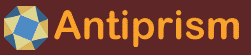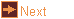# to_nfold - change the rotational symmetry of a polyhedron

 Usage    |    Examples    |    Notes

## Usage

```

Usage: to_nfold [options] fraction [input_file]

Generalise an axial model by changing its rotational symmetry. Read a model,
in OFF format, with an m-fold rotational axis on the z-axis, and create a
new model, generally non-planar, with the same relative connections, but
with an n-fold axis instead. fraction is given as n, or n/d (n and d
integers). Vertices of a face originally separated by x/m of a turn around
the z-axis will be separated by xd/n of a turn in the final model. The new
model will be symmetrically coloured using colours from the base model. If
input_file is not given the program reads from standard input.
to_nfold is based on an idea by Bruce R. Gilson.

Options
-h,--help this help message (run 'off_util -H help' for general help)
--version version information
-x <idxs> vertex index numbers, separated by commas, the rings including
these vertices will be rotated 180 degrees before processing
and rotated back afterwards
-p <args> transform ring of vertices of base model, and suppress normal
to_nfold processing. Arguments are two or three numbers
separated by commas: vertex index number (specifies the ring),
the scale-xy factor (ring radius), and an optional translation-z
(ring height). Can be used multiple times. Index numbers are
preserved.
-o <file> write output to file (default: write to standard output)

```

## Examples

Increase rotational symmetry, e.g. pentagonal prism to hexagonal prism
```   to_nfold 6 pri5 | antiview
```

Decrease rotational symmetry, e.g. pentagonal prism to triangular prism
```   to_nfold 3 pri5 | antiview
```

Change rotational symmetry with a fraction, the denominator introduces a step, e.g. pentagonal prism to {7/2}-prism
```   to_nfold 7/2 pri5 | antiview
```

Change the basic step of a model, e.g. pentagramic dipyramid to pentagonal pyramid (2*3 = 1 mod(5))
```   to_nfold 5/3 dip5/2 | antiview
```

Open an edge, taken as a digon, e.g. convert snub disphenoid to (non-Johnson) snub square antiprism
```   to_nfold 4 j_snub_disphenoid | antiview
```

Close a polygon down to a digon, e.g. convert snub square antiprism to (non-Johnson) snub disphenoid
```   to_nfold 2 j_snub_square_antiprism | antiview
```

Convert a polyhedral torus
```   unitile2d -s t | off_trans -R 90,0,0 | to_nfold 11/2 | antiview
```

A 5/2 crossed-antiprism has oblique faces that cycle the axis, and the result of regular processing does not produce a crossed-antiprism, but the crossed-antiprism form can be preserved with option -x
```   antiview ant5/3
to_nfold 7 ant5/3 | antiview
to_nfold 7 ant5/3 -x 0 | antiview
```

Uncross a crossed-antiprism (the fraction parameter is required, but not used)
```   antiview ant5/3
to_nfold 2 ant5/3 -p 0,-1 | antiview
```

Dimple a ring of vertices of a snub-antiprism (the fraction parameter is required, but not used)
```   antiview snu4
to_nfold 2 snu4 -p 10,0.2 | antiview
```

## Notes

### Algorithm

First the cyclic symmetry of the model, Cn_from, is found. An arbitrary sector of angular width 2π / n_from is considered, and this sector is scaled by rotation to have angular width 2π · d_to / n_to. This determines the new vertex positions unambiguously.

The faces are determined by considering each set of faces which are equivalent under Cn_from symmetry, and then choosing a minimal chain of edges that will repeat by this symmtry to recreate the edges of this set of faces. The chain is transformed, edge by edge, to match the final symmetry and step. It is then repeated by the final symmetry into one or more circuits, which are the final faces.

To transform each edge, the original central angle is considered positive if the symmetry axis lies in the sweep of this edge and the last, otherwise negative, but if the edge intersects the symmetry axis (180 degree edge) then its sign is ambiguous. In this case, if the sum of angles with the adjoining edges is positive then the sign is taken as negative, and vice versa. If the sum is zero, then the sign is chosen, arbitrarily.

### Issues

• If a model has faces that meet at a 180 degree edge in the base model then they may not meet in the final model.
• Some polygons may have a face that shares the axial symmetry but will not divide into valid edge chains, e.g. a rectangular bow-tie quadrilateral has 4 edges and 2-fold symmetry, but a chain of two of its edges cannot be repeated by 2-fold symmetry to recreate the original bow tie.
• Some models have an axial fractional polygon, or a form that can be identified with one (e.g. a polyhedral tube might follow the path of pentagram edges). The step of a polygon tends to be preserved when applying to_nfold, and it may be that the apparent final model step will divide the final n_to. Such models are not constructible, and will lead to a broken final model, likely with edges from a vertex onto itself, e.g. to_nfold 3 pri7/3.
• Faces that slant and are intersected by the axis may lead to skew polygons that wrap the axis several times. This may not be the desired result, however, it is correct for the algorithm. e.g. to_nfold 7 ant5/3. It may be possib;e to correct this with option -x.

to_nfold is based on an idea by Bruce R. Gilson.